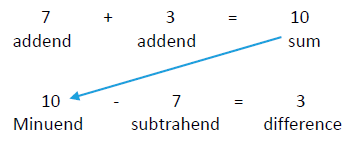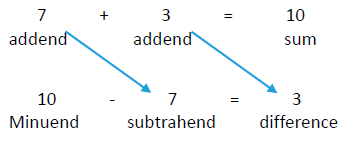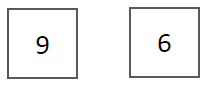# Subtraction

### What is Subtraction?

Subtraction should not be defined as “take away.” So, the question becomes: If subtraction is not take away, then what is it?

The answer is actually very simple when we relate subtraction to addition:

Subtraction is finding a missing addend in an addition statement.

I know. That doesn’t sound so simple, but hopefully the following discussion will make it clearer.

Because subtraction is based on addition, let’s look at some basics of addition first.

We have the addition statement, 7 + 3 = 10.

Now let’s assume that one of the addends is not known, like this

1) 7 + _ = 10

What number is 10 – 7?

The answer: It’s the number you add to 7 to get 10, which of course is 3.

You use subtraction to find an unknown addend when you know one addend and the sum.

When we talk about subtraction, we change the terminology (for some reason), which inadvertently helps to obscure the simple relationship between addition and subtraction.As illustrated above, the number that is the “sum” in addition becomes the “minuend” in subtraction.The “addends” in addition become the “subtrahend” and the “difference” in subtraction.

What’s up with that?

It would be much simpler to say:

• When you know two addends, you add them to find the sum.
• When you know one addend and the sum, you subtract to find the other addend.

(This is one of many cases in math where the terminology we use is so isolated to very small segments of content - “silos” - that we shoot ourselves in our collective feet when it comes to teaching the underlying concepts.)

There’s another way we can define subtraction that means the same thing as the “missing addend” definition.

Subtraction is finding the difference between two numbers.

The “difference” in subtraction is by definition one of the addends in an addition statement - it’s the addend we don’t know. It’s the number you add to a known addend to get a known sum.

So what does this say about teaching the concept of subtraction?

One way to think about subtraction is that it’s “finding a missing part of a number,” where the “number” is the minuend aka, the sum, and the “missing part” is the “unknown addend.”

In order to appreciate this definition, students need to understand that every number is made up of other numbers. Subtraction is just finding one of the numbers that make up another number, the minuend aka, the sum.

What does it mean to say that numbers are composed of other numbers? It’s a fundamental property of numbers. It’s related to what is called the “cardinality” of a number. And what is cardinality?

If you count a group of 8 objects, each count from 1 up to 8 means that you have defined a larger group.

When you point to the first object and say “1,” there’s only 1 item in the group. When you point to the second object and say “2,” you’re not labeling that item, you’re labeling the size of the group that contains that item and the first one you counted. When you count “3,” you’re not labeling that item, you’re describing the size of the group that contains that item, and the two you counted before. And so on.

In other words, each count does not label the item you point to when you count. It labels the size of the group that includes all the items you’ve counted up to that point. That’s the idea we label “cardinality.”

That leads to this conclusion. You can’t have a group of 8 unless you have a group of 7. And you can’t have a group of 7 unless you have a group of 6, and so on. In other words, all whole numbers (with the exception of 0 and 1) are made up of other whole numbers.

This is a fundamental concept that students must acquire in order to understand addition and subtraction. Of course, there’s no reason to teach them to call this “cardinality.” They just need to acquire and use the concept.

This topic is typically referred to as the issue of the “composition and decomposition of numbers.”

Now, how is this related to addition and subtraction?

When you add, you know the “quantity” (cardinality) of two different, non-overlapping groups.

One way to add them is to put the two groups together and count them all, starting with 1 and including all the items in both groups. But that’s a lot of work in many situations. That’s why addition was invented.

For example, here are two boxes.Each box contains some apples, and the number in each box is conveniently written on the outside of the box. (Either you’ve counted these groups in advance, or someone else has counted them and written the numbers on the boxes for you.) There are 9 apples in one box and 6 apples in the other box. For some reason, we want to know how many apples are in the two boxes combined.

One way we could do this is to put these two groups together and count them all. In other words, we find the cardinality of the combined group.

Or, if you have already “committed to memory” the fact that 9 + 6 is 15, or you have learned a strategy to derive the sum, you can use addition.

All you’re doing is “mentally” putting the two groups together (but not physically putting them together, which is a topic for later). We know, after thousands of years of humans repeating this process that the outcome is always 15.

Our ancestors did all the work for us. All we have to do is remember what they learned though centuries of experience. 9 and 6 more is always 15, regardless of what kind of groups the numbers represent. And we don’t have to count all the objects to find this out.

When we listen to our ancestors and recall that fact, we know that the number of apples in the two boxes in all is 15. No work, just a little recall from memory.

Now let’s change this situation to subtraction.Here, we don’t know how many apples are in the second box, but we know that there are 15 in the two boxes combined. We write it like this:

9 + _ = 15

This is where we use subtraction. We know that the number in each box is an addend, and together they make up the number in the sum, which is 15.

We also know that the number “15” has other numbers embedded in it – it’s composed of other numbers (because of cardinality). In this case, we know that one of the “components” of 15 is 9. What we want to know is what the other component is. That’s the number in the second box.

To find the number in the second box (the unknown component of 15?), we subtract. We write the subtraction like this:

15 - 9 = _

In other words, we find the missing addend, the number in the second box. To solve the subtraction problem, we ask the question (mentally) “what number do we add to 9 to get 15?”

Again, we don’t have to do any work. We just rely on what our ancestors have passed on to us. The answer to this question is 6, no matter what kind of things the numbers refer to.

So, the question occurs to me – why in the world have so many intelligent, educated people over the past 100+ years at least, defined subtraction as “taking away?” In this example, there’s nothing to indicate a process of “taking away,” unless you engage in some mental gymnastics (which is not a good idea for teaching kids).

It’s a little strange when you look at it objectively. The real answer is complex, but the simple answer is, “it’s convenient.”

The main reason is that subtraction is an abstract process, like most math topics, so we usually teach it by “grounding it” in experience with physical objects, like building blocks. We have kids “model” the mathematical operations.

But there is a tremendous downside to this approach. The problem is that this single situation that can easily be modeled does not convey the full extent of the concept being taught. Another way to say this is that kids learn from what we don’t say or do as much as they learn from what we do say and do.

Learning subtraction is a classic case of this happening.

Subtraction is used in situations that vary widely. When we teach what subtraction means using a single representation, like “taking away” things, kids unconsciously limit their internalized definition to those limited situations.

They do what is called “undergeneralizing.” That means they assume subtraction is a more limited concept than it really is. When they learn that subtraction “means” only “take away,” they have a difficult time dealing with the fact that in the vast majority of situations in which subtraction is used, nothing is “taken away.”

If we are to overcome this problem, we must design ways of representing the concept of subtraction so that it accurately, and completely, represents the range of situations to which it can be applied. This is the only way to have kids fully understand the concept of subtraction.

See others in this series:

Click below for other Resources